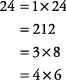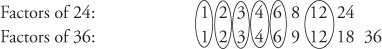## Factors

As mentioned earlier,
factors of a number are whole numbers that when multiplied together yield the number.

##### Example 1

What are the factors of 10?

10 = 2 × 5

and 10 = 1 × 10

So the factors of 10 are 1, 2, 5, and 10.

##### Example 2

What are the factors of 24?Therefore, the factors of 24 are 1, 2, 3, 4, 6, 8, 12, and 24.

#### Common factors

Common factors are those factors that are the same for two or more numbers.

##### Example 3

What are the common factors of 6 and 8?1 and 2 are common factors of 6 and 8.

Note: Some numbers may have many common factors.

##### Example 4

What are the common factors of 24 and 36?Thus, the common factors of 24 and 36 are 1, 2, 3, 4, 6, and 12.

#### Greatest common factor

The greatest common factor (GCF) is the largest factor common to two or more numbers.

##### Example 5

What is the greatest common factor of 12 and 30?Notice that, although 1, 2, 3, and 6 are all common factors of 12 and 30, only 6 is the greatest common factor.